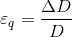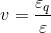## Transverse contraction

Deformation of a solid body. Under the impact of a tensile or compressive force, the body responds with an elongation Δl in the direction of the force and with a reduction or increase in its diameter by Δd in perpendicular direction to the force.Thus, transverse contraction is the decrease in diameter occurring in tensile testing as a result of the test rod’s elastic elongation (eq. 1):

Eq. 1:Εq = transverse contraction; D = original diameter of the test rod in mm; ΔD = change in diameter in mmIn this context, the ratio between transverse contraction and expansion ε (linear expansion) is referred to as Poisson’s ratio (transverse contraction ratio) ν (sometimes also µ) (eq. 2):

Eq. 2:It is also defined as the ratio of the relevant change in thickness Δd/d to the relative change in length Δl/l under the impact of an external force (Fig. 1).The transverse contraction ratio for flake graphite cast iron is 0.26; for nodular graphite cast iron it is 0.28 to 0.29. It is non-dimensional material constant.